# Get Worksheet Name from Formula – Excel

This tutorial will demonstrate how to get a sheet name with a formula in Excel.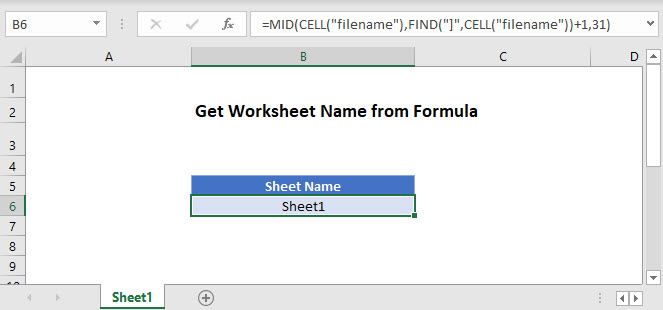## Get Sheet Name

In Excel there isn’t any one function to get the sheet name directly. But you can get a sheet name using VBA, or you can use the CELL, FIND, and MID functions:

``=MID(CELL("filename"),FIND("]",CELL("filename"))+1,31)``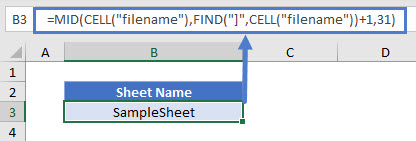Let’s go through the above formula.

### CELL Function

We can use the CELL Function to return the file path, name, and sheet by inputting “filename”.

``=CELL("filename")``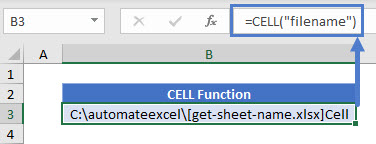To get the current worksheet’s name, you can use the function with or without the optional reference argument, referring to any cell on the current tab.

``=CELL("filename",A1)``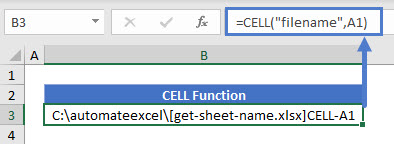You can also get information about any other worksheet by referring to a cell on that sheet.

``=CELL("filename",AnotherSheet!A1)``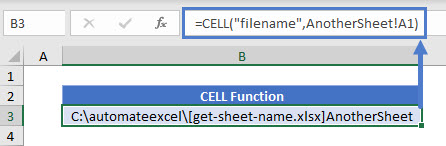### FIND Function

As shown above, the CELL Function returns the file path, name, and worksheet. We don’t need the path or workbook name, so we need to isolate the sheet name.

First use the FIND Function to determine the position of the character immediately before the sheet name (which is always “]”).

``=FIND("]",B3)``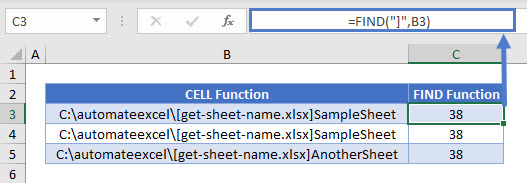## MID Function

Since we have the last character position before the worksheet name, we can now use the MID Function to extract the characters after that position.  Our sheet name starts at the position found above + 1, but we don’t know how long the sheet name is. However, we do know the maximum length of a worksheet name (31), and we can use that in the MID Function:

``=MID(B3,C3+1,31))``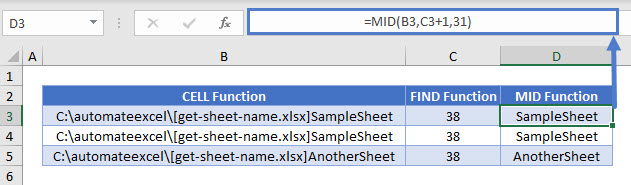Putting this all together yields our original formula:

``=MID(CELL("filename"),FIND("]",CELL("filename"))+1,31)``### Excel Practice Worksheet

Practice Excel functions and formulas with our 100% free practice worksheets!